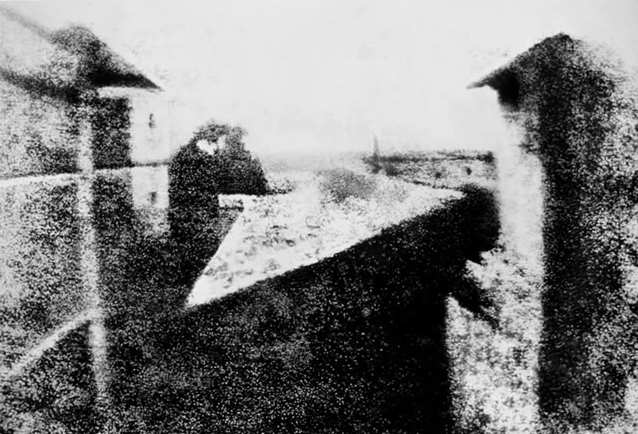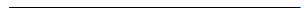Search:

# The birth of photography

## The first photographers: Niépce and DaguerreNiépce (1765-1833) and Louis-Jacques-Mande Daguerre (1787-1851)

## The discovery of Niepce (1820)

Niepce was trying to fix the images on the back window of a camera obscura. First, as others before him, he put there a paper coated with silver chloride. $2Ag^+Cl^-$ $\longrightarrow$ $2Ag$ $+$ $Cl_2$ (Simplified Equation) He obtained "negatives" due to the appearance of black silver in places that were illuminated. But these "photos" had a very short life, since the silver chloride on initially unexposed areas darkened rapidly in the light! Finally, he obtained the first real real photo with a tin plate coated with bitumen, which had been exposed to light for 8 hours:Niépce's first photo : A heliograph

In fact, the bitumen solidifies by oxidation under the influence of light and the non-solidified bitumen can be removed by a solvent

## The discovery of Daguerre (1838)

Here's the process (slightly changed later): - Metallic silver is deposited by oxidation-reduction on a copper plate by treating the copper with a solution containing silver ion $Ag^+$: $Cu$ $-$ $2e^-$ $\longrightarrow$ $Cu^{2+}$ $2Ag^+$ $+$ $2e^-$ $\longrightarrow$ $2Ag$$Cu$ $+$ $2Ag^+$ $\longrightarrow$ $2Ag$ $+$ $Cu^{2+}$ - The plate is polished . - It is exposed to iodine (or bromine) vapor in a dark room to produce a yellowish layer of silver iodide: $2Ag$ $-$ $2e^-$ $\longrightarrow$ $2 Ag^+$ $I_2$ $+$ $2e^-$ $\longrightarrow$ $2I^-$$2Ag+$ $+$ $I_2$ $\longrightarrow$ $2Ag^+I^-$ (Simplified Equation) - This layer is subjected to the action of the light by exposure in the camera obscura. $2Ag^+I^-$ $\longrightarrow$ $2Ag$ $+$ $I_2$ (Simplified Equation) - The image is revealed: For this, the plate is placed in a mercury-containing box heated to 60 degrees. Mercurial vapor produce a white amalgam with the silver formed by light exposure. - The image is fixed by dissolving the unaltered iodide (or bromide) with a thiosulfate solution (Daguerre took a concentrated solution of sea salt), to prevent any subsequent action of light. $Ag^+I^-$ $+$ $2 S_2O_3^{2-}$ $\longrightarrow$ $Ag(S_2O_3)_2^{3-}$ $+$ $I^-$ (see →   the process)Daguerre's first photo: A daguerreotype